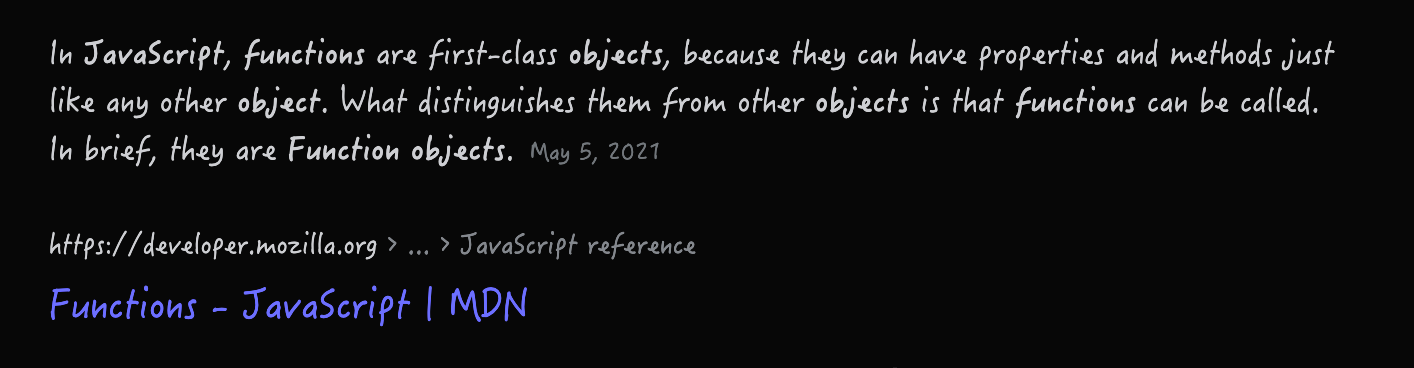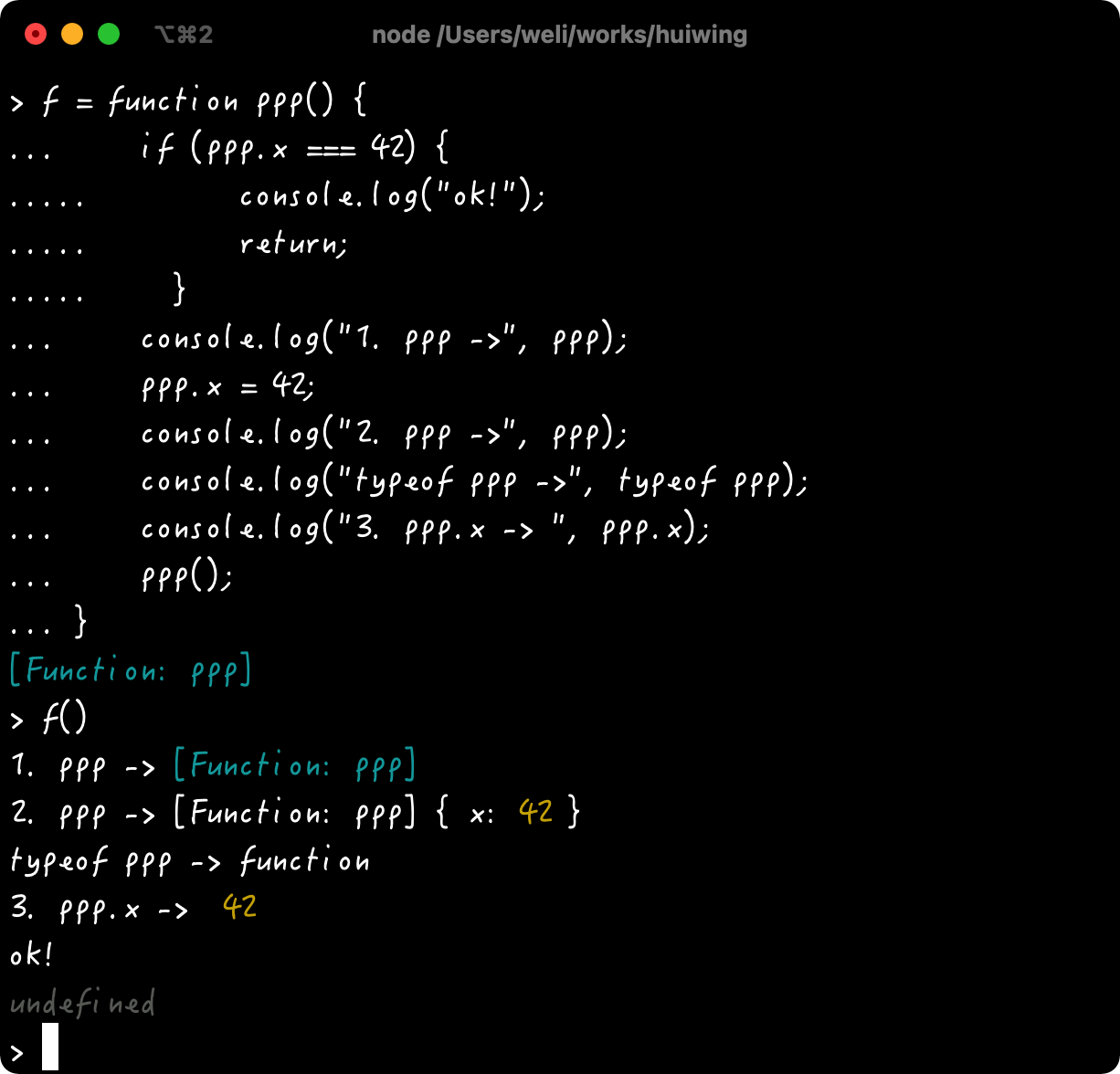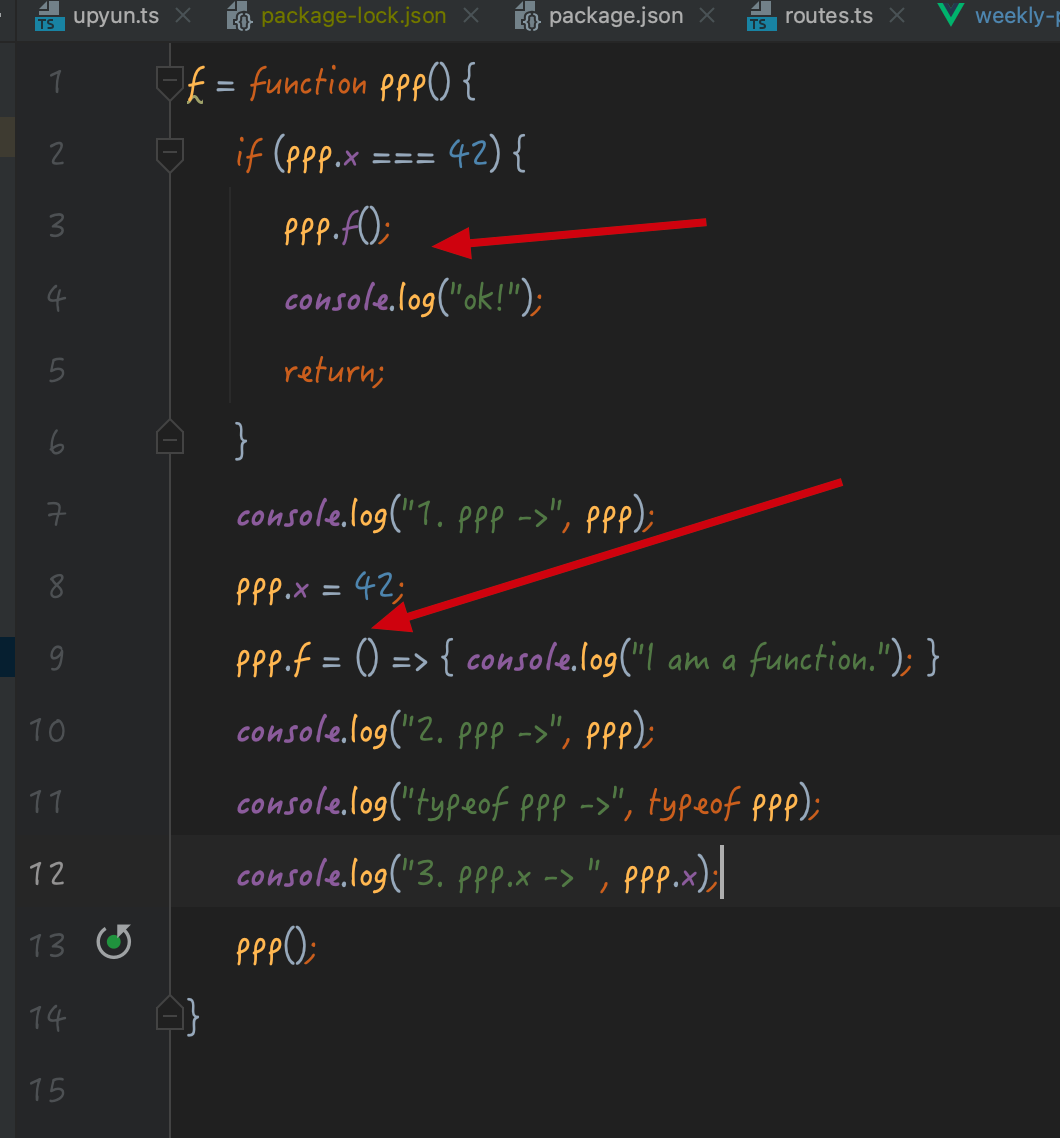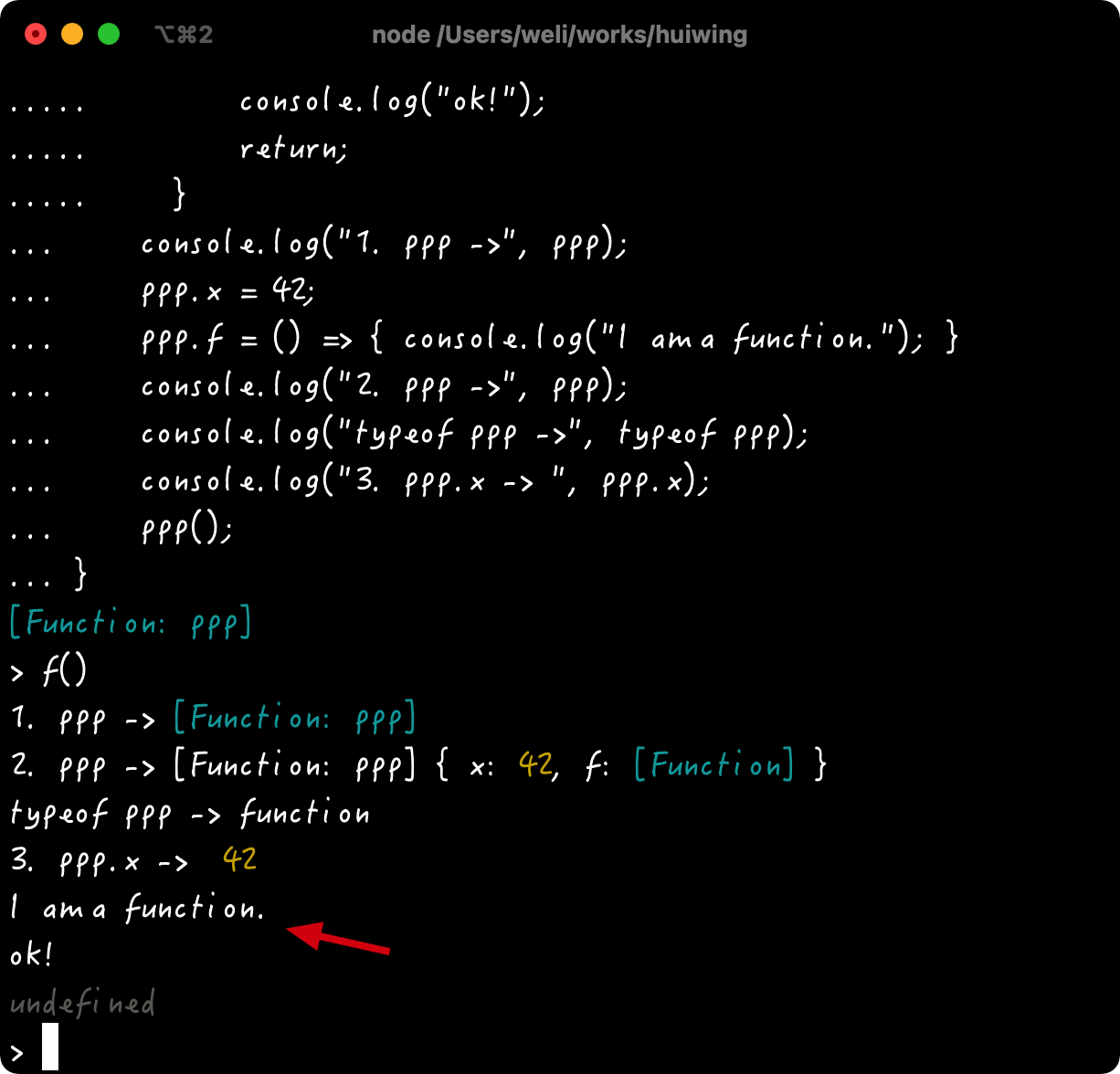Javascript / Function is an object

In JavaScript web page it says functions are first-class objects:Here is the code to set a property x to the function:

f = function ppp() {
if (ppp.x === 42) {
console.log("ok!");
return;
}
console.log("1. ppp ->", ppp);
ppp.x = 42;
console.log("2. ppp ->", ppp);
console.log("typeof ppp ->", typeof ppp);
console.log("3. ppp.x -> ", ppp.x);
ppp();
}

In above code we set a property x to ppp function itself, and call itself recursively. Here is the running result:From above we can see the property x is set, and function is called recursively.

In addition we can also set a function to a function itself:In above code we can see a f() function is added to ppp() function itself. Running the above code will get the result in below:From above we can see the ppp.f() function can be called properly.